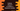# Python program to check if a number is a disarium number or not## Python program to check if a number is a disarium number or not:

In this post, we will learn how to check if a number is a disarium number or not using Python. This program will take one number as input from the user and print one message i.e. if it is a disarium number or not.

Before we start to write the program, let’s understand what is a disarium number and the algorithm that we will use.

### What is a Disarium number:

A number is called a disarium number if the sum of the digits raised to the power of their positions is equal to the number itself.

The position starts from 1, i.e. the position of the leftmost digit is 1, the second digit from the left is 2 etc.

For example, 175 is a disarium number. Because, if we calculate the sum of the digits raised to the power of their positions, it will be 1^1 + 7^2 + 5^3, which is 175, i.e. the number itself.

### Method 1: By calculating the sum using a loop:

Let’s use a loop to pick the rightmost digit of the number, find the power of that number and add that value to a final sum variable. At the end of the loop, we will compare the number with the sum.

Let’s take a look at the program:

``````def find_sum(no, l):
sum = 0
while no > 0:
last_digit = no % 10
sum = sum + int(last_digit ** l)
l = l - 1
no = int(no/10)
return sum

given_num = int(input('Enter a number: '))

if given_num == find_sum(given_num, len(str(given_num))):
print(f'{given_num} is a Disarium number')
else:
print(f'{given_num} is not a Disarium number')``````

Here,

• find_sum method is used to find the sum of all digits of the number raised to the power of its position.
• This method takes two parameters.The number and the length of the number, i.e. the count of digits in the number.
• Inside this method, we are initializing the variable sum as 0 to hold the final sum.
• The while loop will run until the value of no, is greater than 0. Inside the loop, we are finding the last digit of the number and adding the value of last_digit ** l to sum. The value of l is decremented by 1 and the last digit of no is removed by dividing it by 10.
• Once the while loop ends, it returns the sum.
• We are checking if the value of the user given number, i.e. given_num is equal to the return value of find_sum or not. If yes, we are printing that this is a Disarium number. Else, we are printing that it is not a Disarium number.

“ If you run this program, it will print output as like below:

``````Enter a number: 89
89 is a Disarium number

Enter a number: 123
123 is not a Disarium number``````

### Method 2: Without converting the number to a string:

We can also read the user input as a string. We can iterate through the characters of the string one by one and find the sum. Let me show you the program:

``````def find_sum(no):
sum = 0
p = 1
for c in no:
sum = sum + pow(int(c), p)
p = p + 1
return sum

given_num = input('Enter a number: ')

if given_num.isdigit() == False:
print('Please enter a valid number !')
else:
if int(given_num) == find_sum(given_num):
print(f'{given_num} is a Disarium number')
else:
print(f'{given_num} is not a Disarium number')``````

Here,

• The input number is stored as a string in the given_num variable.
• The first if statement checks if a user-input number is a valid number or not. If it is not a valid number, it prints one message to the user. Else, it finds the sum.
• The find_sum method takes only one parameter, i.e. the string value entered by the user.
• The sum is initialized as 0.
• p is initialized as 1. This is the power value.
• By using the for loop, we are iterating through the characters of the string one by one.
• For each character, we are converting it to an integer by using the int() method and we are finding the power by using the pow method. This value is added to the sum variable.
• The value of p is incremented by 1 after each iteration of the for loop.
• Once the loop ends, it returns the value of sum.
• Based on the return value, it prints a message.

If you run this program, it will print output as like below: